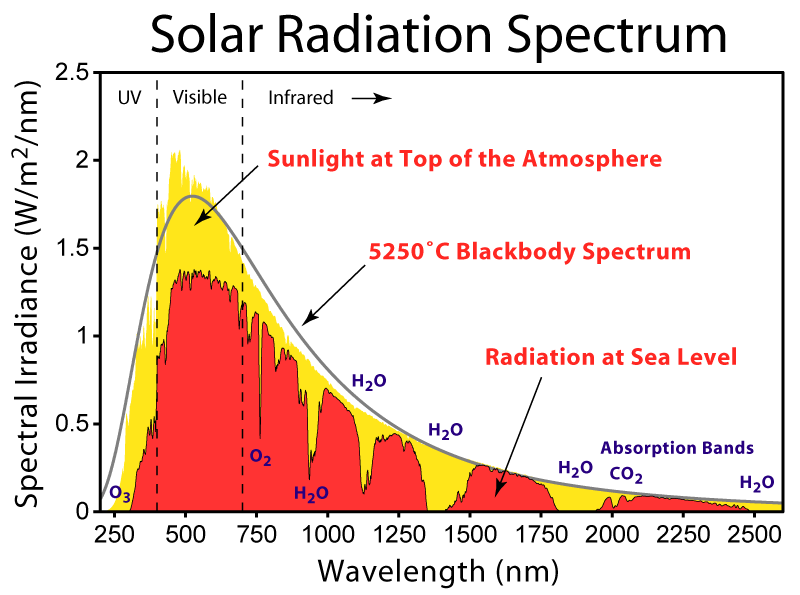# Section 6¶

In :
from IPython.display import Latex
import matplotlib.pyplot as plt
%matplotlib inline
import numpy
from matplotlib import animation, rc
from IPython.core.display import HTML
from IPython.display import HTML
from ipywidgets import *
from scipy.integrate import odeint
from IPython.display import Image


## Equilibrium Temperature of the Earth¶

#### How Hot is the Sun?¶

We learned that the Sun can be modeled as a Blackbody. If we measure the radiance of the Sun at the top of the atmosphere, we get the following curve:

In :
PATH = "/Users/hunteradams/Documents/PhD Semester 5/Sections/"
Image(filename = PATH + "Solar_Spectrum.png", width=600, height=600)

Out:We see that the peak of this Blackbody curve is at about 501.5 nm, or 0.5015 $\mu m$. Remember Wien's Law?

\begin{align} \lambda_{max} = \frac{2898 \mu m \cdot T}{T} \end{align}

Solving for $T$:

\begin{align} T &= \frac{2898}{\lambda_{max}} \end{align}

Substituting the measured $\lambda_{max}$:

\begin{align} T &= \frac{2898}{0.5015} = 5778K \end{align}

#### How Much Energy/Time/Area is the Sun Emitting?¶

Remember Stephan-Boltzmann?

\begin{align} F &= \sigma T^4 \end{align}

where $\sigma = 5.67 \times 10^{-8} \frac{\text{W}}{\text{m}^2\text{K}^4}$, and $F$ has units of energy/time/area, or $\frac{W}{m^2}$. So, the energy per unit time (W) per unit area ($m^2$) emitted by the Sun is:

\begin{align} F &= \left(5.67 \times 10^{-8}\right)\left(5778^4\right)\\ &= 6.31 \times 10^7 \frac{W}{m^2} \end{align}

#### How Much Total Energy/Time (Luminosity)?¶

Recall that to get total energy/time (Watts), we multiply the energy/time/area by the surface area of the star:

\begin{align} L &= 4\pi R^2 \sigma T^4 \end{align}

We know that the Sun has a radius of the Sun is $6.97 \times 10^8$ meters. So, the luminosity is:

\begin{align} L &= 4\pi \left(6.97\times 10^8\right)^2 \left(6.31 \times 10^7\right)\\ &= 3.85 \times 10^{26} W \end{align}

#### How Much Energy/Time at the Earth?¶

Recall that this total energy gets distributed on the surface of a sphere with radius equal to the distance to the Sun.

\begin{align} P_{Earth} &= \frac{L}{4\pi R_{E\rightarrow S}^2} \end{align}

#### The distance from the Earth to the Sun is about $1.49 \times 10^{11}$ meters. Thus, the solar flux at the Earth is:¶

\begin{align} P_{Earth} &= \frac{3.85 \times 10^{26}}{4\pi \left(1.49 \times 10^{11}\right)^2}\\ &= 1370 \frac{W}{m^2} = S_0 \end{align}

This is known as the Solar Constant.

#### How Much Energy is Coming Into the Earth from the Sun?¶

We know that there is 1370 $\frac{W}{m^2}$ coming from the Sun at one AU away. How much of the Earth's total surface area intercepts this energy? The energy is intercepted by the disk that faces the Sun. So...the total incoming energy is give by:

\begin{align} E_{incoming} &= \pi R_E^2 S_0 \end{align}

But! Not all of this energy is absorbed! In fact, only about 70 percent is absorbed (the rest is reflected). This equation is then augmented as:

\begin{align} E_{incoming} &=(1-A) \pi R_E^2 S_0\\ &= 0.7 \pi R_E^2 S_0 \end{align}

For now, let's not substitute values into this equation. We'll see why in a moment.

#### How much Energy is Emitted by the Earth?¶

What does this energy do when it hits the Earth? It heats it up. If we assume that the Earth is heated up pretty uniformly, then it can be approximated by a blackbody. Remember that from Stephen-Boltzmann, the $\frac{W}{m^2}$ emitted by a blackbody is given by:

\begin{align} F &= \sigma T^4 \end{align}

So, as before, the total energy emitted is given by:

\begin{align} L_{Earth} &= E_{outgoing}= 4\pi R_E^2 \sigma T^4 \end{align}

Note that energy was absorbed only on the disk ($\pi R_E^2$), and is emitted over the entire surface area of the Earth ($4\pi R_E^2$).

#### What is the Equilibrium Temperature of the Earth?¶

Equilibrium means that the total incoming energy is equal to the total outgoing energy. The Earth isn't getting hotter or colder when it's in equilibrium, it's at a temperature where these energies are balanced. How do we enforce this condition? We set the incoming energy equal to the outgoing energy:

\begin{align} E_{incoming} &= E_{outgoing}\\ 0.7 \pi R_E^2 S_0 &= 4\pi R_E^2 \sigma T^4 \end{align}

Cancelling terms:

\begin{align} 0.7 S_0 &= 4\sigma T^4 \end{align}

Solving for $T$:

\begin{align} T &= \left(\frac{0.7S_0}{4\sigma}\right)^{\frac{1}{4}} \end{align}

Substituting:

\begin{align} T &= \left(\frac{0.7\times 1370}{4 \times 5.67 \times 10^{-8}}\right)^{\frac{1}{4}}\\ &= 255K \end{align}

What is this in Celcius? $255-273 = -18C \approx 0F$. Super cold.

## Why Isn't the Earth 0F?¶

The Earth is not 0F because of the Greenhouse Effect. What is the wavelength of incident light from the the Sun? Wien's Law:

\begin{align} \lambda_{max} &= \frac{2898}{T}\\ &= \frac{2898}{5778}\\ &= 0.6 \mu m = 600 nm \end{align}

What is the wavelength of light re-emitted by the Earth?

\begin{align} \lambda_{max} &= \frac{2898}{T}\\ &= \frac{2898}{255}\\ &= 11.36 \mu m = 11,360 nm \end{align}

That's a much longer wavelength. Remember what we learned about absorption and emission spectra? Specific molecules will absorb and re-emit light at very specific wavelengths, and it will allow light that isn't at those wavelengths to pass unimpeded. Which direction does the light get re-emitted? All directions.

So the atmosphere, which has C02, methane, etc., allows short-wavelength light from the Sun to pass unimpeded. However, it absorbs and re-emits light at longer wavelengths. Because that light is re-emitted in all directions, some is sent back down to Earth, warming it more.

In [ ]: